Tapping into mathematics

Start this free course now. Just create an account and sign in. Enrol and complete the course for a free statement of participation or digital badge if available.

Free course

# 6 Large and small numbers

## 6.1 Scientific notation

Understanding how your calculator displays and handles very large and very small numbers is important if you are to interpret the results of calculations correctly. This section focuses on a way of representing numbers known as scientific notation.

Before you start put your calculator into the float mode, so it will display up to about 10 digits and return to the home screen ready to do some calculations.

What answer would you expect if you square 20 million? How many zeros would the answer have? If you do this on paper, you should end up with 4 followed by 14 zeros. However your calculator might not be able to display 14 figures, if it is set to 10 figures. So input this calculation into your calculator and see what it does:

20000000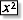You will probably get something like 4e14. The notation e14 should be read as 10 to the power 14 (or 10 to the 14) which means fourteen 10s multiplied together. It is written as 1014. So the calculator answer of 4e14 is 4 × 1014, which is short for 400000000000000.

The number 14, that is the power of 10, is also called the exponent and means the number of 10s which are multiplied together. The letter E in 4e14 is used to mean exponent.

Now instead of 20 million squared, what would 90 million squared be? What would you expect? Try it on your calculator and see.

Since 20 million squared was 4 × 1014, you might expect that 90 million squared would be 81 × 1014.

However, the calculator shows a different answer. Try it and see.

The calculator's answer of 8.1e15 or 8.1 × 1015 is in fact the same number as 81 × 1014.

Both these numbers represent 8 100 000 000 000 000.

However, when a number is written using a scientific notation the convention is to use a number between 1 and 10 before the exponent. So here, instead of 81 × 1014, the number 8.1 is used with an extra power of 10.

Another way of interpreting 8.1 × 1015 is to imagine that the decimal point in the number 8.1 should be moved 15 places to the right, filling in as many zeros as necessary.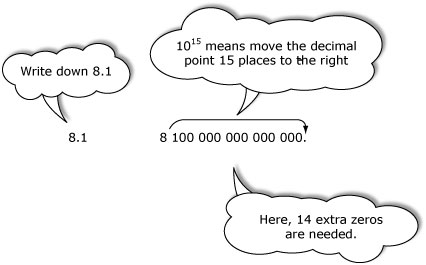### Exercise 10: The sky's the limit

• (a) The speed of light measured in meters per second, is about 300 million (300 000 000) meters per second. What is this number in scientific notation?

• (b) Einstein's famous equation says that E = mc2, the energy E (in joules) associated with a mass of m kilograms is equal to m multiplied by c squared, where c is the speed of light (in meters per second). Using the value of c given in your answer to part (a), work out the value of E in scientific notation for a mass of 1 kilogram.

• (c) The distance from the Earth to the Sun is about 149 million kilometres. What is this number in scientific notation?

• (d) What is the largest number your calculator can handle? For example, enter any number and keep squaring. How far can you go before the calculator displays an error message?

(a) 300 000 000 = 3 × 108

(b)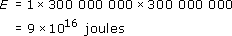(c)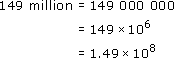(d) The TI-84 calculator cannot handle numbers with exponents of 100 or more. Notice that the calculator produces a message saying ERR:OVERFLOW.

Small numbers very close to zero can also be expressed in scientific notation. For example, enter a simple starting value such as 4.3 and then repeatedly divide by 10. This can be done by pressing

4 . 3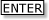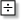1 0And so on.

Watch carefully how the numbers go: 4.3, .43, .043, .0043, etc. Then at some point the display will jump into scientific notation. Different calculators use different methods of displaying scientific notation. Look in your calculator handbook to check how your calculator displays scientific notation and compare it with that used by the TI-84, which gives .00043 as 4.3e4.

The e4 part means that the decimal point in the number 4.3 should be moved four places to the left, which requires the insertion of some additional zeros.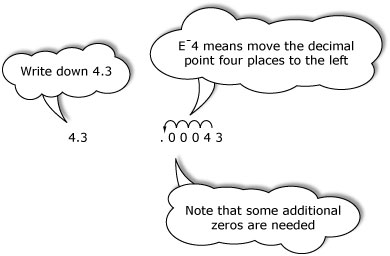What happens to the result if you keep dividing by 10? You should see the exponent change from 4 to 5 to 6, and so on. As the negative exponent gets larger, the corresponding decimal number gets smaller.

As with large numbers, the notation e4 should be read as ‘10 to the power 4’ (or simply ‘10 to the minus 4’).

Do not worry about negative powers of 10 for the moment. Just think of the notation as a way of writing down small numbers where the negative sign reminds you that the decimal point must be moved to the left. Here is another example.

The calculator display 3.75 e6 means the same as 3.75 × 10−6.

So the procedure is as follows.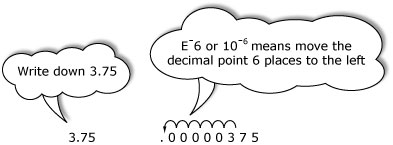The smallest number above zero that the calculator can handle is 1.0 e99. Any smaller numbers are simply recorded as zero on your calculator. However, even though your calculator cannot handle numbers smaller than 1.0 e99 (that is, 10−99), you can imagine numbers as small as you like.

### Exercise 11: Small is?

• (a) What are the following numbers in scientific notation?

• 0.1     0.0045     0.000 000 6

• (b) Convert these numbers back to conventional decimal form.

• 3.78 × 104     8.91e7     0.7 × 103

(a) 0.1 = 1 × 10−1        0.0045 = 4.5 × 10−3        0.000 000 6 = 6 × 10−7

(b) 3.78 × 10−4 = 0.000 378        8.91e7 = 0.000 000 891       0.7 × 10−3 = 0.0007

### Brain stretcher: 1+1=2e0

Using the MODE menu, change the setting in the top line from Normal to Sci.

Carry out a range of different calculations.

When would this calculator setting be helpful for you?

(Do not forget to return to the Normal setting when you have finished exploring this question)

Now imagine that all the numbers which your calculator is capable of displaying are set out in a line. A version of what the calculator number line might look like is shown in Figure 1, but note that it is not drawn to scale.

Zero is at the centre of the line. To the right of zero lie all the positive numbers up to 1099. To the left of zero lie all the negative numbers up to 1099. Around zero are all the numbers between 10−99 and 10−99, which the calculator treats as zero. It is worthwhile spending some time studying Figure 1 and being clear about what it means.

Consider the following questions and try to answer them for yourself before reading the comment.

• What is the distinction between the number 1099 and 10−99?

• This number line has not been drawn anything like to scale. What might it look like if it were? If the interval on the number line between 0 and 1 000 000 000 occupied, say 3 cm on the page, how far away would you have to place the value 1099 using the same scale? Would you expect an answer in meters, kilometres or in astronomical numbers such as the distance from the Earth to the Sun.

It is important to recognise the difference between negative numbers and numbers with negative powers. For example, 1099 is a very large negative number (written out fully it would be 1 followed by 99 zeros) which lies a long way to the left of zero on the number line. In contrast 10−99 is a very small positive number which is very close to zero (this time the full version would look like 0.000000…00001, with 98 zeros between the decimal point and the 1). This tiny number would lie just to the right of zero on the number line.

MU120_4MCAL